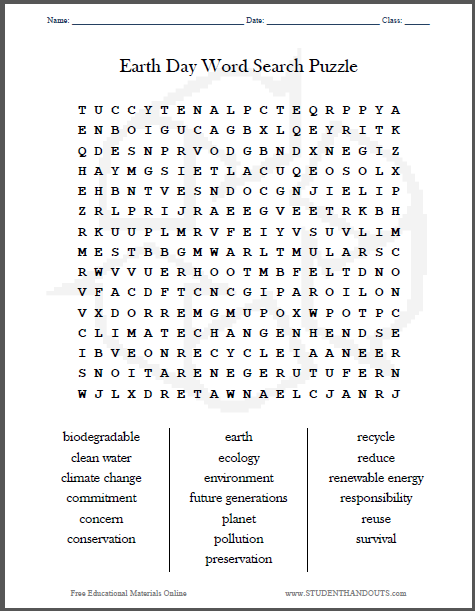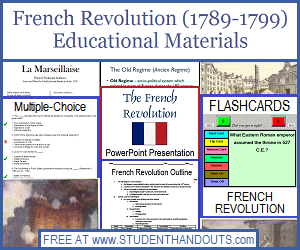Earth Day Word Search Puzzle | Student Handouts
 Share the learning joy!
Earth Day Word Search PuzzleThis word search puzzle contains 19 terms related to Earth Day: biodegradable, clean water, climate change, commitment, concern, conservation, earth, ecology, environment, future generations, planet, pollution, preservation, recycle, reduce, renewable energy, responsibility, reuse, survival. Click here to print. For more of our free educational materials related to Earth Day, click here.T + C + Y T E N A L P + + E + R P + Y +
+ N + O + G + C A + + + L + E + R + T +
+ + E + N + R V O + + B + D + N E + I +
+ + + M + S I E + L A + U + E O S + L +
E + + + T V E + N D O C + N + I E + I +
+ R + + R I + R A E E G V + E T R + B +
+ + U U + + M R V + E I Y + S U V + I +
+ + S T + + G M + A R L + + U L A + S C
+ + + + U E + H O O T + B + E L T + N O
+ + + + D F T + N C + I + A R O I + O N
+ + + O + R + M + + + + O + W P O + P C
C L I M A T E C H A N G E N + E N + S E
+ B + E + N R E C Y C L E + + + N + E R
S N O I T A R E N E G E R U T U F E R N
+ + + + + R E T A W N A E L C + + + R +